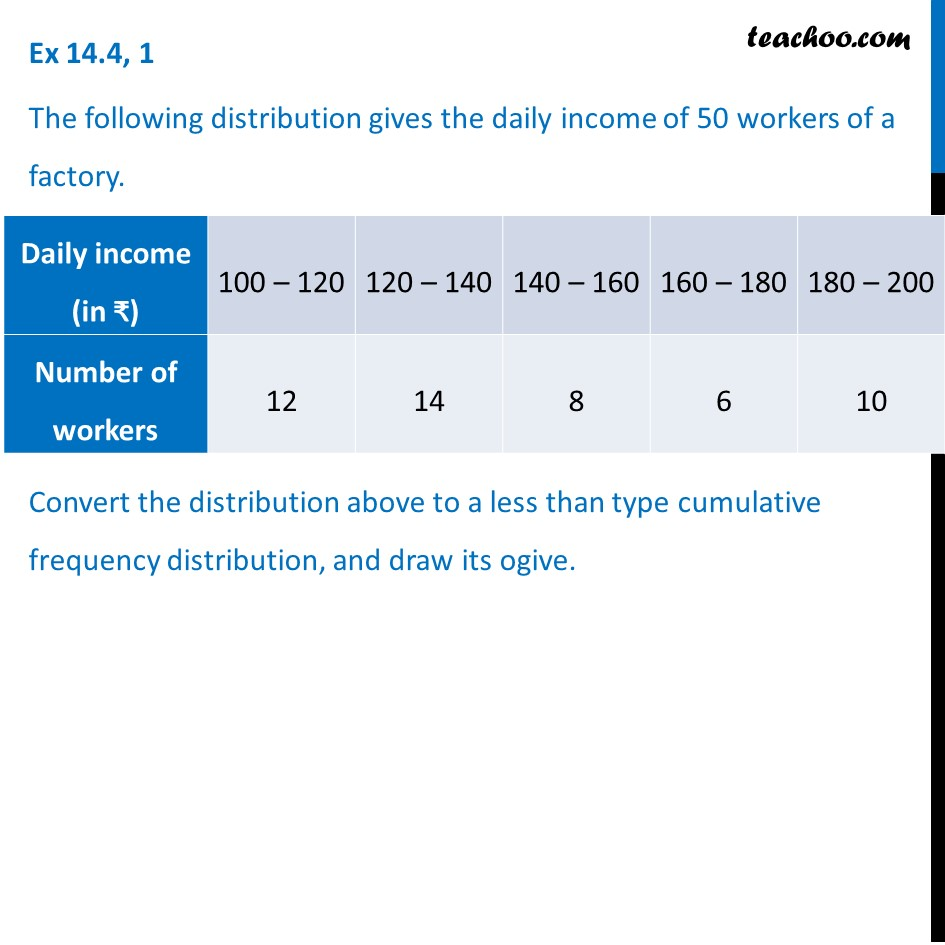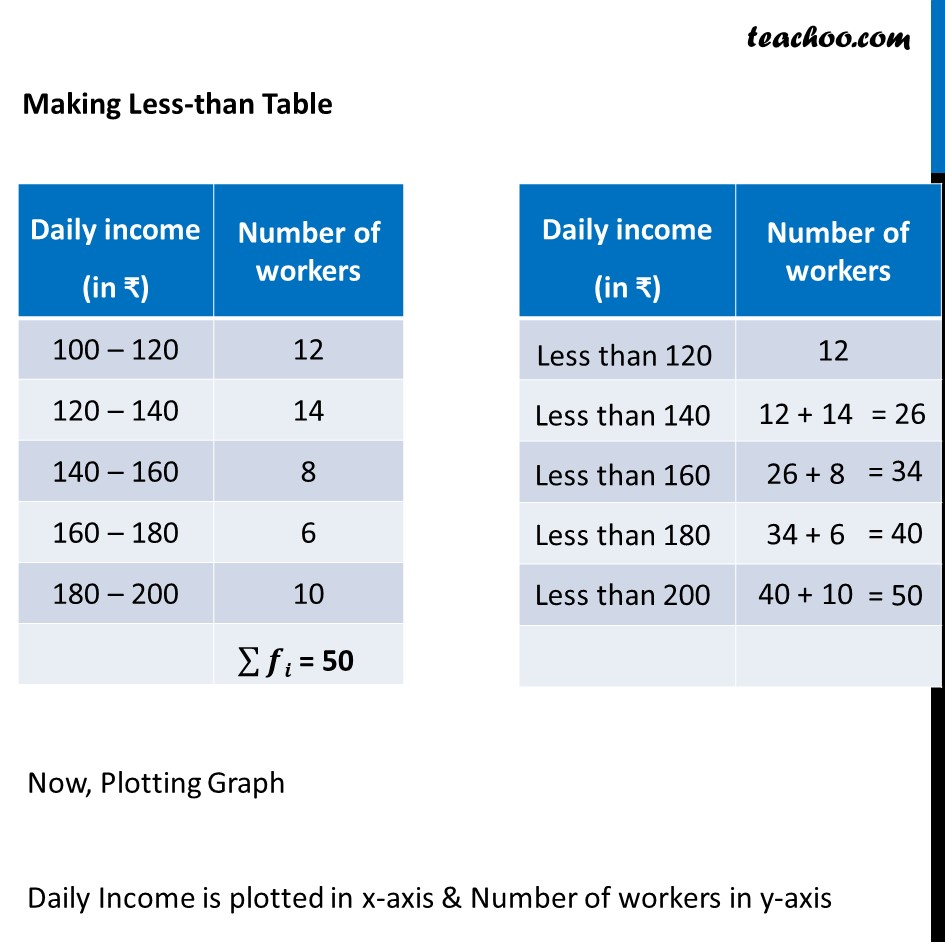1. Chapter 14 Class 10 Statistics (Term 2)
2. Serial order wise
3. Ex 14.4

Transcript

Ex 14.4, 1 The following distribution gives the daily income of 50 workers of a factory. Convert the distribution above to a less than type cumulative frequency distribution, and draw its ogive. Making Less-than Table Now, Plotting Graph Daily Income is plotted in x-axis & Number of workers in y-axis Less than 120 12